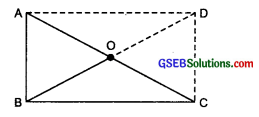# GSEB Solutions Class 8 Maths Chapter 3 Understanding Quadrilaterals Ex 3.4

Gujarat Board GSEB Textbook Solutions Class 8 Maths Chapter 3 Understanding Quadrilaterals Ex 3.4 Textbook Questions and Answers.

## Gujarat Board Textbook Solutions Class 8 Maths Chapter 3 Understanding Quadrilaterals Ex 3.4

Question 1.
State whether True or False.
(a) All rectangles are squares.
(b) All rhombuses are parallelograms.
(c) All square are rhombuses and also rectangles.
(d) All squares are not parallelograms.
(e) All kites are rhombuses.
(f) All rhombuses are kites.
(g) All parallelograms are trapeziums.
(h) All squares are trapeziums.
Solution:
(a) False
(b) True
(c) True
(d) False
(e) False
(f) True
(g) True
(h) TrueQuestion 2.
Identify all the quadrilaterals that have:
(a) Four sides of equal length.
(b) Four right angles.
Solution:
(a) Square as well as rhombus have four sides of equal length.
(b) Squares, as well as rectangles, have four right angles.

Question 3.
Explain how a square is:

2. a parallelogram
3. a rhombus
4. a rectangle

Solution:

1. A square is a 4-sided figure, so it is a quadrilateral.
2. The opposite sides of a square are equal and parallel, so it is a parallelogram.
3. All the sides of a square are equal, so it is a rhombus.
4. Each angle of a square is a right angle, so it is a rectangle.Question 4.

1. bisect each other
2. are perpendicular bisectors of each other
3. are equal

Solution:

1. The diagonals of the following quadrilaterals bisect each other: Parallelogram, rectangle, square, rhombus
2. The diagonals are perpendicular bisectors of the following quadrilaterals: Square and rhombus
3. The diagonals are equal in case of: Square and rectangle

Question 5.
Explain why a rectangle is a convex quadrilateral.
Solution:

1. All the angles have measures less than 180°.
2. Both diagonals lie wholly in the interior of the rectangle.

∴The rectangle is a convex quadrilateral.Question 6.
ABC is a right-angled triangle and O is the mid-point of the side opposite to the right angle. Explain why O is equidistant from A, B and C. (The dotted lines are drawn additionally by to help you.)
Solution:
Produce BO to D such that BO = OD. Joining CD and AD, we get a quadrilateral ABCD in which opposite sides are parallel.
∴ ABCD is a parallelogram.∵∠ABC = 90°
∴ABCD is a rectangle
Since, diagonals of a rectangle bisect each other, i.e., O is the mid-point of BD and AC.
∴ O is equidistant from A, B and C